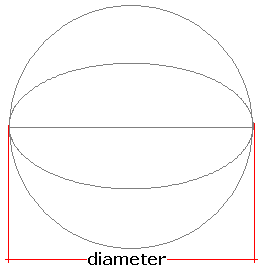Spike's Calculators

# Sphere Volume and Surface Area

Calculate the volume of a sphere. Results include the surface area in square units and the sphere's arc length (circumference).This calculator works for any measurement unit. For example, if you calculate inches, inches will have to be used in all the length fields, and the result of the areas will be square inches; the volume results in cubic inches and length measurements in the starting unit inches.

### Sphere

Diameter #
Decimal Precision #

#### Results:

 Diameter of the Sphere # Volume of the Sphere #³ Sphere Surface Area #² Arc Length # Surface to Volume Ratio sa:vol

#### Calculation

• enter the sphere diameter
• decimal precision, number of digits after the decimal point

#### Results

• the diameter of the sphere (starting unit)
• the volume of the sphere (unit cubed)
• surface area (unit squared)
• arc length, the circumference (starting unit)
• surface area to volume ratio (decimal)
##### Formula
```V = 4/3Π(D/2)³
C = 2Π(D/2)
SA = 4Π(D/2)²
s/v = SA/V
where V is the volume of the sphere
C the circumference
SA the surface area
s/v the surface area to volume ratio
Π = 3.14159265
D the diameter
```